The  TetrahedronFigure 1

Each side of the tetrahedron is in green. We will refer to the side or edge of the tetrahedron as ‘ts.’
The tetrahedron has 6 sides, 4 faces and 4 vertices. In Figure 1 the base is marked out in gray: the triangle BCD. Each of the faces is an equilateral triangle.
From   The Equilateral Triangle  we know that:
Area BCD =.

Now we need to get the height of the tetrahedron, AH.Figure 2

From  The Equilateral Triangle  we know that:
BH =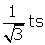.

Now that we have BH, we can find  AH, the height of the tetrahedron. We will call that  h.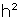=.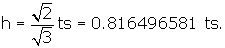= 1/3 * area BCD * h =What is the surface area of the tetrahedron?  It is just the sum of the areas of its 4 faces.
We know from above that the area of a face is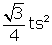.
The total surface area of the tetrahedron =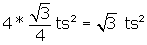.
We have found the volume of the tetrahedron in relation to it's side.

Since all 4 vertices of the tetrahedron will fit inside a sphere, what is the relationship of the side of the tetrahedron to the radius of the enclosing sphere

It's easier to see the radius of the enclosing sphere if we place the tetrahedron inside a cube: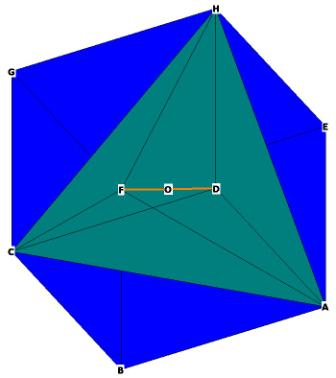Figure 3

The 4 vertices of the tetrahedron are H,F,C,A.
The 4 faces of the tetrahedron in this picture are: CFH,CFA,HFA, and at the back, HCA.
(The vertex D is a part of the cube, not the tetrahedron).
The vertices of the cube all touch the surface of the sphere. The diameter of the sphere is the diagonal of the cube FD.
In this analysis and the ones following, we will assume that all of our polyhedra are enclosed within a sphere of radius = 1. That way we will be able to accurately assess the relationship between all of the 5 regular solids.
The radius of this sphere is OF = OD = 1. How does the side of the tetrahedron relate to the radius of the sphere?
The side of the tetrahedron is the diagonal of the cube, as can be seen in Figure’s 3 and 4.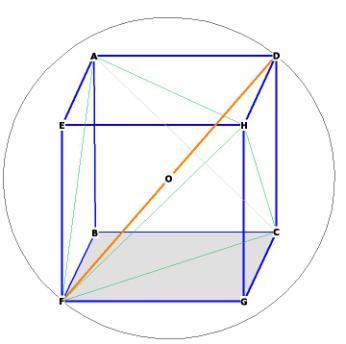Figure 4
FC is the side of the tetrahedron, DC is the side of the cube, FD is the diagonal of the cube and the diameter of the sphere enclosing the cube and the tetrahedron.
By the Pythagorean Theorem,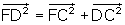.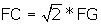, DC = 1.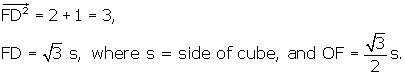So FG(side of cube), FC(side of tetrahedron), and FD(diameter of enclosing sphere)
have a 1,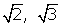relationship.

What is the relationship between the side of the tetrahedron and the radius of the enclosing sphere? We want to know the relationship between OF and FC.. FD = 2 * OF, so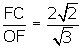. Therefore,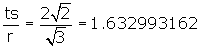.

So we write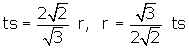.

Where is the center of mass of the tetrahedron? We have marked it as O in the preceding figures. The centroid is just the distance O to any of the tetrahedron vertices, or the radius of the enclosing sphere. The center of mass of the cube is also the center of mass of the tetrahedron; as is evident from Figure 3 and Figure 7, every vertex of the tetrahedron is a vertex of the cube.
We already have OF, or r, which is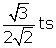. If we compare this distance to the height of the tetrahedron, we get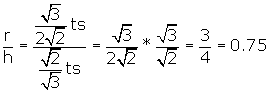Therefore AO = 3 * OH.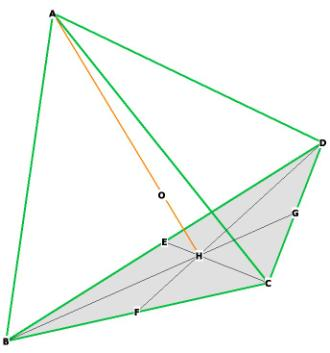Figure 1, repeated.

Compare this to the equilateral triangle, where the relationship is 2 to 1.
What is the central angle of the tetrahedron? The central angle is the angle from one vertex, through the centroid O, to another vertex. This can best be seen from Figure 3.

vertex                          vertex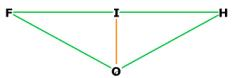centroid
Figure 5
We calculate everything in terms of the side of the tetrahedron.
OF = OH = radius of enclosing sphere. FH = ts.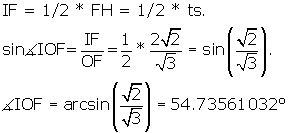The central angle FOH = 2 * <IOF.
Central angle = 109.47122064What is the surface angle of the tetrahedron? The surface angle is that angle made by the angles within the faces. Since each face of the tetrahedron is an equilateral triangle, the surface angle = 60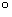What is the dihedral angle of the tetrahedron?
The dihedral angle is the angle formed by the intersection of 2 planes: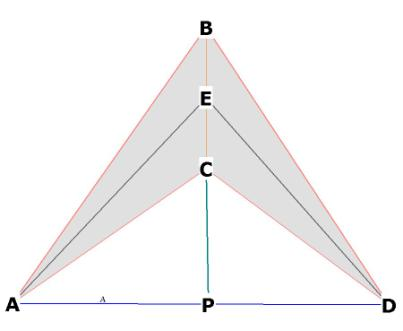Figure 6
BAC and BDC are 2 intersecting faces of the tetrahedron. E is at the midpoint of BC.
AE and DE are lines that go through the middle of each face and hit E.
The dihedral angle is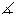AED.
The triangle EPD is right.PED = 1/2AED by construction.
AD is the side of the tetrahedron s, so PD = ½ * ts
ED, the height of the tetrahedron face =.
sin(PED) = PD/ED =.PED = arcsin (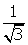) = 35.26438968AED = 2 *PED, Dihedral angle = 70.52877936Now we will calculate the distances between the centroid and
1) the middle of one of the faces (OJ)
2) the midpoint of one of the sides (OI)
3) any vertex (OH)Figure 7, showing  centroid distances.

OF = distance from centroid to a vertex, in this case, F,
OI = distance from centroid to middle of a side, in this case, the side FH
OJ = distance from centroid to middle of a face, in this case, face ACF,

The 4 faces are CFH, CFA, HFA and HCA as before. The middle of face CFA is J. The midpoint of the side of the tetra FH is at I.
We have already figured out OF = OC = OA = OH, the distance from the centroid to any vertex. This is, as you recall,.
Let's get OI first.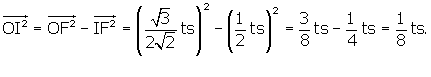OI =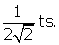The triangle FJO in Figure 7 is right becauseOJF is right*. This will enable us to get the distance OJ.

From we know that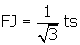.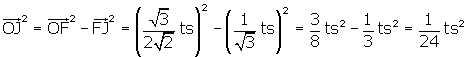,
OJ =.

To get a good idea of relative distances,
re write OF  as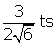and OI as.
Then the relationship between OJ, OI and OF is 1,, 3.

___________________________________________

·        Note to teachers: if you build an octahedron and a rhombic dodecahedron off the octahedron faces (use the Zometool), and then attach a tetrahedron off one of the octahedron faces, the centroid of that tetrahedron will be the vertex of the rhombic dodecahedron. In fact, the distance from the centroid of the tetrahedron to each of it’s vertices is the edge length of the rhombic dodecahedron! Nature is not only beautiful, but also logical and consistent.

Tetrahedron Reference Tables
(included in the book)